I have created YouTube playlists by difficulties.

Problem:

Given a string S, find the number of different non-empty palindromic subsequences in S, and return that number modulo 10^9 + 7.

A subsequence of a string S is obtained by deleting 0 or more characters from S.

A sequence is palindromic if it is equal to the sequence reversed.

Two sequences A_1, A_2, ... and B_1, B_2, ... are different if there is some i for which A_i != B_i.

Example 1:

Input:
S = 'bccb'
Output: 6
Explanation:
The 6 different non-empty palindromic subsequences are 'b', 'c', 'bb', 'cc', 'bcb', 'bccb'.
Note that 'bcb' is counted only once, even though it occurs twice.


Example 2:

Input:
Output: 104860361
Explanation:
There are 3104860382 different non-empty palindromic subsequences, which is 104860361 modulo 10^9 + 7.


Note:

• The length of S will be in the range [1, 1000].
• Each character S[i] will be in the set {'a', 'b', 'c', 'd'}.

Idea:

DP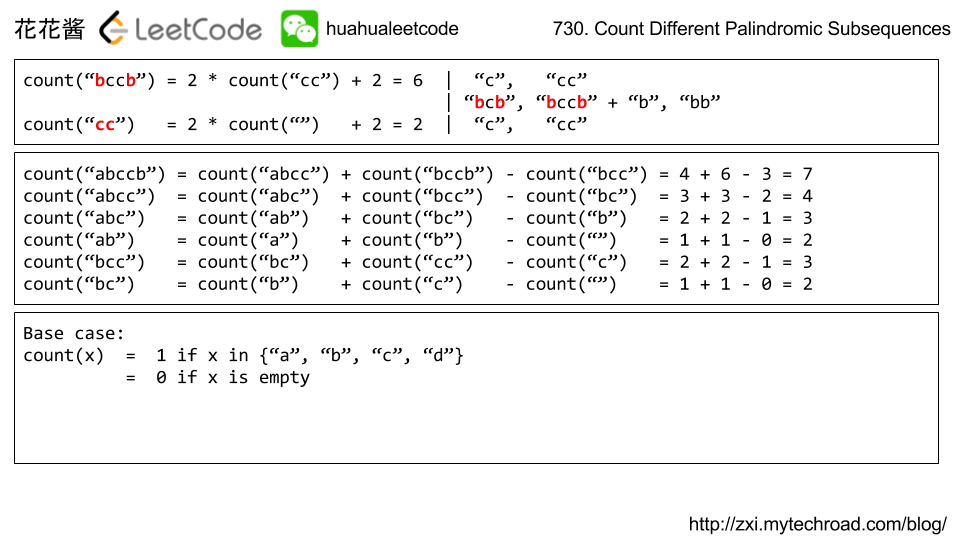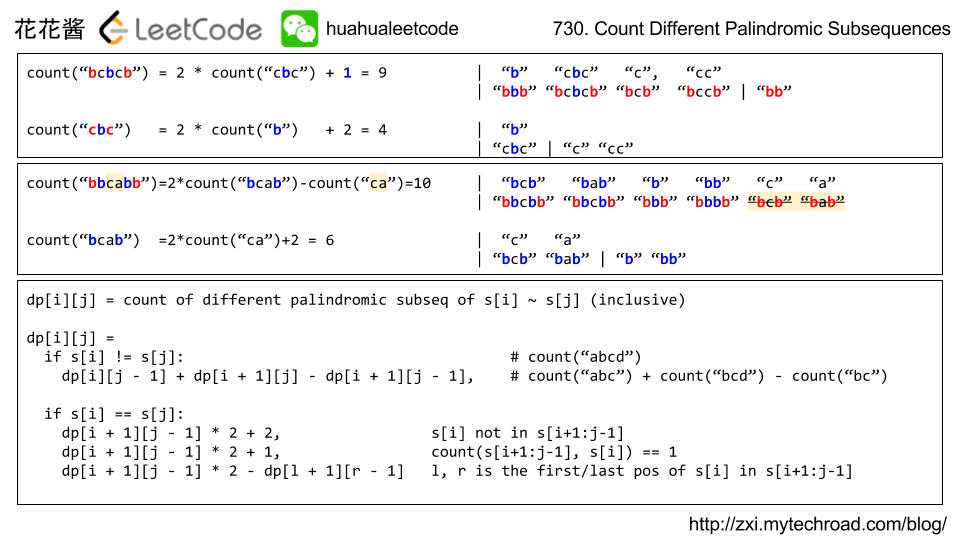# Solution 1: Recursion with memoization

Time complexity: O(n^2)
Space complexity: O(n^2)

# Solution 2: DP

## Pyhton

Related Problems:

Problem:

Implement a MyCalendarTwo class to store your events. A new event can be added if adding the event will not cause a triple booking.

Your class will have one method, book(int start, int end). Formally, this represents a booking on the half open interval [start, end), the range of real numbers x such that start <= x < end.

triple booking happens when three events have some non-empty intersection (ie., there is some time that is common to all 3 events.)

For each call to the method MyCalendar.book, return true if the event can be added to the calendar successfully without causing a triple booking. Otherwise, return false and do not add the event to the calendar.

Your class will be called like this: MyCalendar cal = new MyCalendar(); MyCalendar.book(start, end)

Example 1:

Note:

• The number of calls to MyCalendar.book per test case will be at most 1000.
• In calls to MyCalendar.book(start, end)start and end are integers in the range [0, 10^9].

Idea:

Brute Force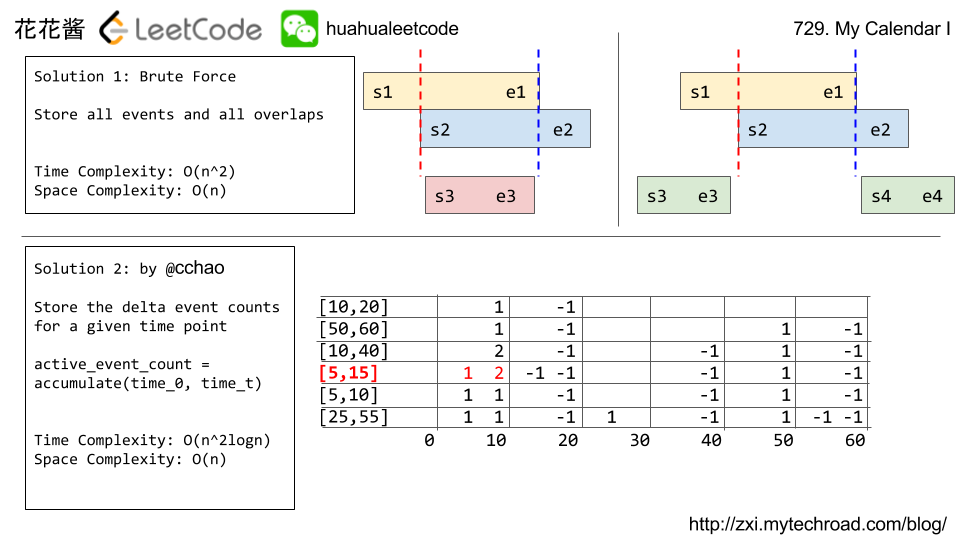Solution1:

Brute Force

Time Complexity: O(n^2)

Space Complexity: O(n)

Java

Python

Solution 2:

Counting

Time Complexity: O(n^2logn)

Space Complexity: O(n)

Related Problems:

Problem:

Implement a MyCalendar class to store your events. A new event can be added if adding the event will not cause a double booking.

Your class will have the method, book(int start, int end). Formally, this represents a booking on the half open interval [start, end), the range of real numbers x such that start <= x < end.

double booking happens when two events have some non-empty intersection (ie., there is some time that is common to both events.)

For each call to the method MyCalendar.book, return true if the event can be added to the calendar successfully without causing a double booking. Otherwise, return false and do not add the event to the calendar.

Your class will be called like this: MyCalendar cal = new MyCalendar(); MyCalendar.book(start, end)

Example 1:

Note:

• The number of calls to MyCalendar.book per test case will be at most 1000.
• In calls to MyCalendar.book(start, end)start and end are integers in the range [0, 10^9].

Idea:

Binary Search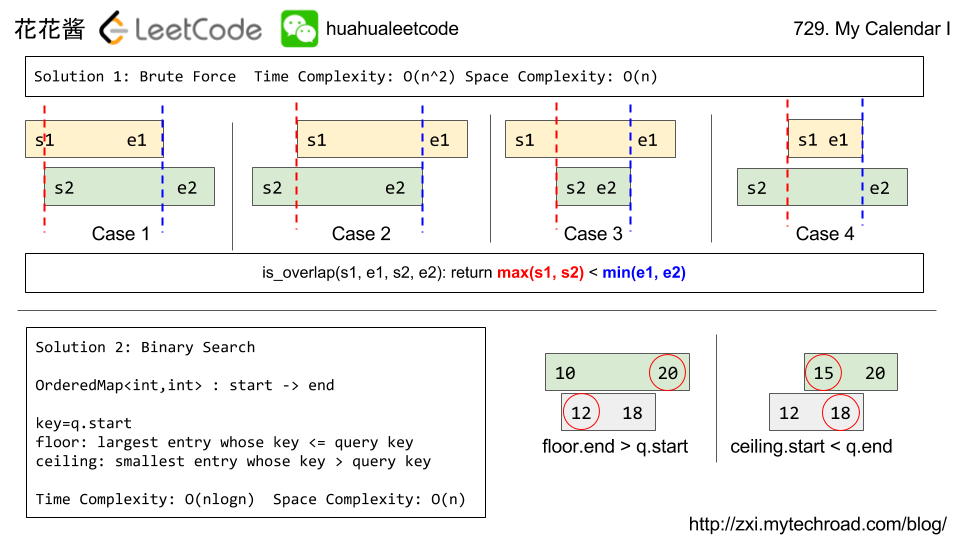Solution1:

Brute Force: O(n^2)

C++

Solution 2:

Binary Search O(nlogn)

C++

Java

Related Problems:

Problem:

self-dividing number is a number that is divisible by every digit it contains.

For example, 128 is a self-dividing number because 128 % 1 == 0128 % 2 == 0, and 128 % 8 == 0.

Also, a self-dividing number is not allowed to contain the digit zero.

Given a lower and upper number bound, output a list of every possible self dividing number, including the bounds if possible.

Example 1:

Note:

• The boundaries of each input argument are 1 <= left <= right <= 10000.

Idea: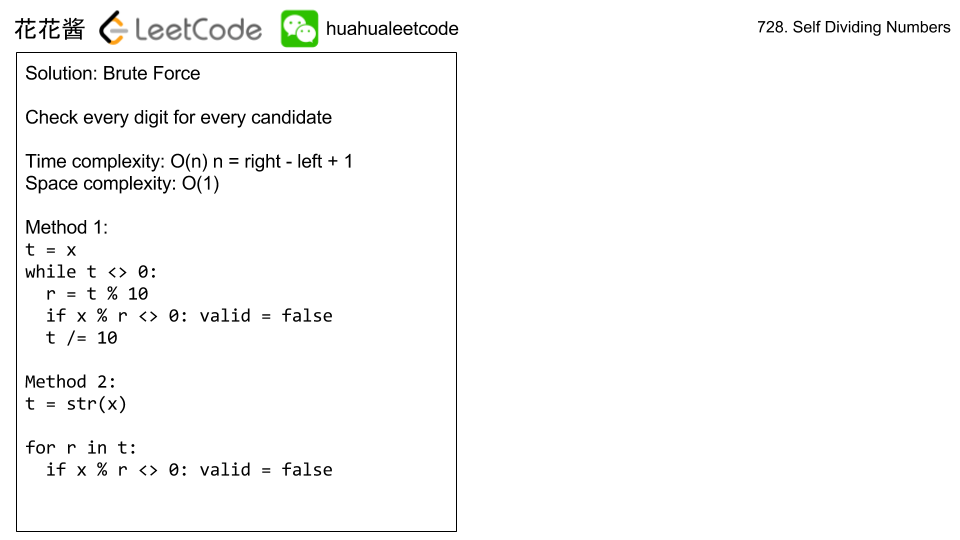Brute Force

Time Complexity: O(n)

Space Complexity: O(1)

Solution:

C++

String

Related Problems:

Mission News Theme by Compete Themes.